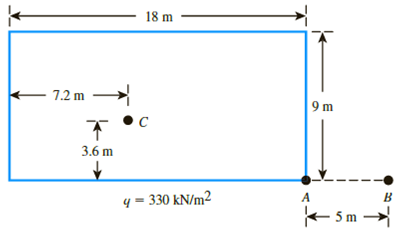Chapter 10, Problem 10.17PPrinciples of Geotechnical Enginee...

9th Edition
Braja M. Das + 1 other
ISBN: 9781305970939

Solutions

Chapter
SectionPrinciples of Geotechnical Enginee...

9th Edition
Braja M. Das + 1 other
ISBN: 9781305970939
Textbook Problem

Refer to Figure 10.47. A flexible rectangular area is subjected to a uniformly distributed load of q = 330 kN/m2. Determine the increase in vertical stress, Δσz, at a depth of z = 6 m under points A, B, and C.Figure 10.47

To determine

Calculate the increase in vertical stress under points A, B, and C.

Explanation

Given information:

The uniformly distributed soil (q) is 330kN/m2.

The depth (z) is 6m.

The length of the rectangular cross section (L) is 18m.

The breadth of the rectangular cross section (B) is 9m.

Calculation:

Calculate the increase in vertical stress (Δσz) using the relation as follows.

Δσz=qI3 (1)

Here, I3 is the influence factor and as a function of m and n.

Stress increase at A:

Calculate the ratio (m) as shown below.

m=Lz (2)

Substitute 18m for L and 6m for z in Equation (2).

m=186=3

Calculate the ratio (n) as shown below.

n=Bz                                                                                                                         (3)

Substitute 9m for B and 6m for z in Equation (3).

n=96=1.5

Refer Table 10.10 “Variation of I3 with m and n” in the Text Book.

Take the value of I3 as 0.225, for the values m of 3 and n of 1.4.

Take the value of I3 as 0.2309, for the values m of 3 and n of 1.6.

Calculate the value of I3, for the values m of 3 and n of 1.5 using interpolation as shown below.

1.61.51.61.4=0.2309I30.23090.2250.5×0.0059=0.2309I30.00295=0.2309I3I3=0.2279

Calculate the increase in vertical stress (Δσz) at point A as shown below.

Substitute 330kN/m2 for qo and 0.2279 for I3 in Equation (1).

Δσz=330×0.2279=75.2kN/m2

Hence, the increase in vertical stress at point A is 75.2kN/m2_.

Stress increase at B:

Sketch the cross section of the loading diagram along point B as shown in Figure 1.

Refer to Figure 1.

Consider the two rectangular section of 23m×9m and 5m×9m.

Consider the rectangular section of 23m×9m.

Calculate the ratio (m) as shown below.

Substitute 23m for L and 6m for z in Equation (2).

m=236=3.83

Calculate the ratio (n) as shown below.

Substitute 9m for B and 6m for z in Equation (3).

n=96=1.5

Refer Table 10.10 “Variation of I3 with m and n” in the Text Book.

Take the value of I3 as 0.2250, for the values m of 3 and n of 1.4.

Take the value of I3 as 0.2260, for the values m of 4 and n of 1.4.

Calculate the value of I3, for the values m of 3.83 and n of 1.4 using interpolation as shown below.

43.8343=0.2260I30.22600.22500.00017=0.2260I3I3=0.2258

Take the value of I3 as 0.2309, for the values m of 3 and n of 1.6.

Take the value of I3 as 0.2320, for the values m of 4 and n of 1.6.

Calculate the value of I3, for the values m of 3.83 and n of 1.6 using interpolation as shown below.

43.8343=0.2320I30.23200.23090.000187=0.2320I3I3=0.2318

Calculate the value of I3(1), for the values m of 3.83 and n of 1.5 using interpolation as shown below.

1.61.51.61.4=0.2318I3(1)0.23180.22580.5×0.006=0.2318I3(1)0.003=0.2318I3(1)I3(1)=0.2288

Consider the rectangular section of 5m×9m.

Calculate the ratio (m) as shown below.

Substitute 5m for L and 6m for z in Equation (2).

m=56=0.833

Calculate the ratio (n) as shown below.

Substitute 9m for B and 6m for z in Equation (3).

n=96=1.5

Refer Table 10.10 “Variation of I3 with m and n” in the Text Book.

Take the value of I3 as 0.1739, for the values m of 0.8 and n of 1.4.

Take the value of I3 as 0.1836, for the values m of 0.9 and n of 1.4.

Calculate the value of I3, for the values m of 0.833 and n of 1.4 using interpolation as shown below.

0

Still sussing out bartleby?

Check out a sample textbook solution.

See a sample solution

The Solution to Your Study Problems

Bartleby provides explanations to thousands of textbook problems written by our experts, many with advanced degrees!

Get Started

If such an e-mail came in, what would be the best action to take?

Principles of Information Security (MindTap Course List)